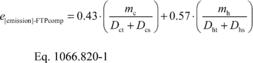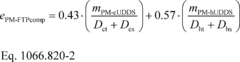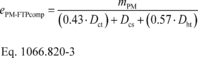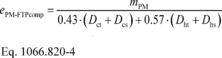# Title 40

## SECTION 1066.820

### 1066.820 Composite calculations for FTP exhaust emissions.

§ 1066.820 Composite calculations for FTP exhaust emissions.

(a) Determine the mass of exhaust emissions of each pollutant for each FTP test interval as described in § 1066.605.

(b) Calculate the final composite gaseous test results as a mass-weighted value, e[emission]-FTPcomp, in grams per mile using the following equation:Where: mc = the combined mass emissions determined from the cold-start UDDS test interval (generally known as bag 1 and bag 2), in grams. Dct = the measured driving distance from the transient portion of the cold-start test (bag 1), in miles. Dcs = the measured driving distance from the stabilized portion of the cold-start test (bag 2), in miles. mh = the combined mass emissions determined from the hot-start UDDS test interval in grams. This is the hot-stabilized portion from either the first or second UDDS (bag 2, unless you measure bag 4), in addition to the hot transient portion (bag 3). Dht = the measured driving distance from the transient portion of the hot-start test (bag 3), in miles. Dhs = the measured driving distance from the stabilized portion of the hot-start test (bag 4), in miles. Set Dhs = Dcs for testing where the hot-stabilized portion of the UDDS is not run.

(c) Calculate the final composite PM test results as a mass-weighted value, ePM-FTPcomp, in grams per mile as follows:

(1) Use the following equation for PM measured as described in § 1066.815(b)(1), (2), or (3):Where: mPM-cUDDS = the combined PM mass emissions determined from the cold-start UDDS test interval (bag 1 and bag 2), in grams, as calculated using Eq. 1066.605-3. mPM-hUDDS = the combined PM mass emissions determined from the hot-start UDDS test interval (bag 3 and bag 4), in grams, as calculated using Eq. 1066.605-3. This is the hot-stabilized portion from either the first or second UDDS (bag 2, unless you measure bag 4), in addition to the hot transient portion (bag 3).

(2) Use the following equation for PM measured as described in § 1066.815(b)(4):Where: mPM = the combined PM mass emissions determined from the cold-start UDDS test interval and the first 505 seconds of the hot-start UDDS test interval (bag 1, bag 2, and bag 3), in grams, as calculated using Eqs. 1066.605-4 and 1066.605-5.

(3) Use the following equation for PM measured as described in § 1066.815(b)(5):Where: mPM = the combined PM mass emissions determined from the cold-start UDDS test interval and the hot-start UDDS test interval (bag 1, bag 2, bag 3, and bag 4), in grams, as calculated using Eqs. 1066.605-6 and 1066.605-7. [79 FR 23823, Apr. 28, 2016, as amended at 81 FR 74214, Oct. 25, 2016]